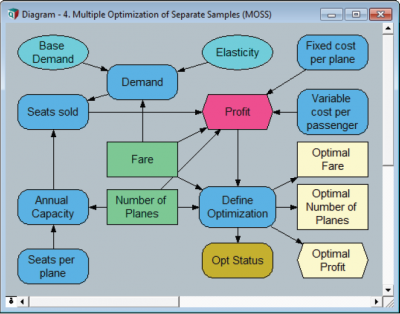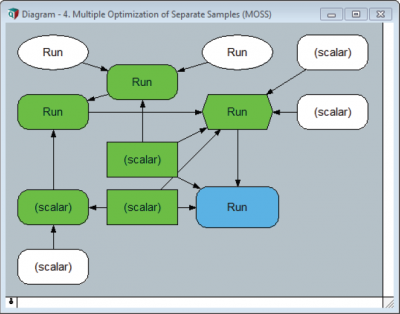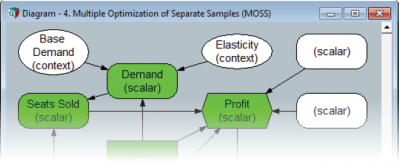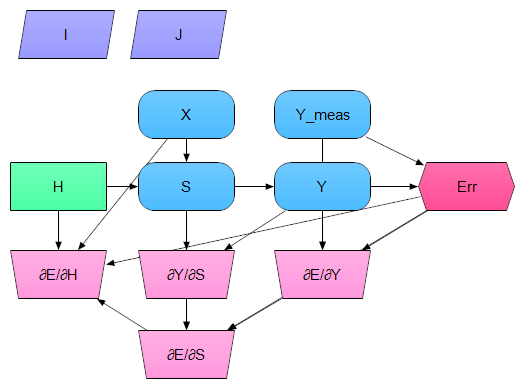# Improving Computational Efficiency of NLPs

## Setting Context

Module 4 of the Airline NLP was the first example in which we used an extrinsic index (Run) with an NLP. Since this was a very simple example with only ten samples, the result appeared pretty much instantly. Improving computational efficiency in this case would be important only to the world’s most ambitious competitive coffee drinking champion. But real world NLPs can be very demanding on processing cycles. The dirty secret of the Module 4 example described above is that it made about ten times as many calculations as it needed to. Since Module 4 is intended to be a proxy for larger models, we need to fix this problem and make it run even faster!

Remember that NLP search algorithms are iterative. The optimizer repeatedly sets new Decision values and re-evaluates every downstream variable, all the way to the Objective value.The influence diagram makes it easy to identify variables that are evaluated repeatedly during the search. These include Annual_Capacity, Seats_Sold, Demand, and of course the Objective: Profit.

If any of these variables contains an extrinsic index (such as Run in this example) Analytica will compute the entire array for each iteration. This includes slices for all elements of Run whether they are needed or not. But Run is an extrinsic index in this version of the Airline model. This means that each element of Run has its own optimization and vice versa. Within a given optimization, the optimizer is interested in only one Run element even though entire arrays are being evaluated repeatedly. The superfluous slices are discarded by the optimizer, and the next iteration starts.

The optional «SetContext» parameter of DefineOptimization allows you to identify nodes for which only a single element of the extrinsic index applies to a given optimization. This avoids the inefficiency described above.To identify the best context variables, let’s look at the same influence diagram in a different light. Nodes have been re-labeled here to show the extrinsic indexes present or “scalar” if none. Iterated quantities (i.e. quantities downstream of Decisions) are colored green.

There are a few basic principles of context setting:

• A context variable will not propagate extrinsic indexes to downstream variables during the optimization.
• You should avoid using variables that are downstream of Decisions. They are repeatedly evaluated and will therefore be only partially effective at improving efficiency.
• Context variables should be as close to the optimization as possible without being downstream of Decisions.
• The set of context variables should include only as many as necessary to prevent propagation of extrinsic indexes to iterated quantities.

In this example, Setting context on Demand would eliminate the Run index from Seats Sold and Profit. But Demand is downstream of a Decision and is therefore NOT the most suitable candidate.Base Demand and Elasticity are the right choices. They are only evaluated once, and together they can eliminate Run from the rest of the model during optimization.

The diagram above shows how the chosen context variables prevent the extrinsic index from being propagated to iterated variables. Iterated variables end up being scalar in terms of their extrinsic dimensions in the context of a single optimization. Outside the optimization, the dimensions of these arrays don’t change.

The following definition will dramatically improve the performance of the Airline NLP Module 4 example:

Variable Opt := DefineOptimization(
Decisions: Number_of_Planes, Fare,
Maximize: Profit,
SetContext: [Base_Demand, Elasticity])

Note
In this example, Run was merely an example of an extrinsic index. The «SetContext» parameter is important for all NLPs that use extrinsic indexes whether they contain uncertain quantities or not.

New to Analytica 5.0

In some cases you might be able to compute the gradients and Jacobians for your NLP explicitly as Analytica expressions, and this can dramatically speed up solve times in some NLPs. Most NLP algorithms estimate the gradient at each point, and use this when decide where to head next during search. This is especially true of the GRG Nonlinear engine, which is a gradient-descent algorithm.

A gradient variable is depicted on an influence diagram as a top-heavy trapezoid, and is used to compute the partial derivative of one variable with respect to another. Each of these reference variables may be multidimensional in general. The Definition of a gradient variable specifies how to compute the gradient, and you as a modeler are responsible for writing this Definition. The Definition of the gradient variable can use the results from other gradient or non-gradient variables in the model. A gradient variable has two attributes not present in other variable types: The Gradient of attribute and the With respect to attribute. Each of these attributes must be populated with the identifier of the corresponding variable. The Gradient of attribute can also contain the identifier of a constraint node.

Consider the following example, which is one layer of a conventional feed-forward neural network. X is indexed by I and J; H by J; and Y and Y_meas by I, and Y_meas does not depend on X, S or Y.Variable S := Sum( H * X, J )
Variable Y := Tanh( Degrees(S) )
Variable Err := Sum( (Y - Y_meas)^2, I)

Several gradient variables of potential interest could be computed as follows:

Gradient dE_dY := 2 * (Y - Y_meas)
GradientOf dE_dY := Err
WithRespectTo dE_dY := Y
Gradient dY_dS := 1 / Cosh(Degrees(S))^2
GradientOf dY_dS := Y
WithRespectTo dY_dS := S
Gradient dE_dS := dE_dY * dY_dS
GradientOf dE_dS := Err
WithRespectTo dE_dS := S
Gradient dE_dH := X * dE_dS
GradientOf dE_dH := Err
WithRespectTo dE_dH := H

The example uses many variables to make the application of the chain rule of derivatives pretty explicit. For example, the chain rule says that

$\displaystyle{ {{\partial E}\over{\partial S}} = {{\partial E}\over{\partial Y}} {{\partial Y}\over{\partial S}} }$

which is indeed the Definition of dE_dS. A separate gradient variable for dS_dH was not included, but had it been it would have been defined as just X; nevertheless, we see this X appear in the definition of dE_dH from the application of the chain rule of differentiation. It is not necessary to have separate gradient nodes -- you could also define dE_dH as X * 2 * (Y - Y_meas) / Cosh(Degrees(S))^2; the example was broken down as such to demonstrate that the result of gradient variables can be used by other gradient variables, which is often convenient.

An optimization that "trains" this neural network is formulated as

DefineOptimization( Decisions:X, Minimize: Err )

and is an NLP. DefineOptimization will automatically locate and use the gradient dE_dH, which it does via the Gradient Of and With respect to attributes. In a training example like this, an explicit gradient reduces training times dramatically.

The following steps are used to create a gradient variable:

1. Create a general variable.
2. In the Object window or Attribute panel, change its class to Gradient.
3. Fill it its GradientOf and WithRespectTo attributes.
4. Fill it its Definition

The gradient variable does not appear on the toolbar, so it is always constructed in this manner.

When computing the gradient $\displaystyle{ \partial C / \partial x }$ of a constraint C such as f(x) <= g(x), your gradient will computed the gradient of the left-hand side minus the right-hand side, i.e., $\displaystyle{ \partial(f(x)-g(x)) / \partial x }$. The same is true for a greater-than constraint or an equality constraint.

When you have a cascaded constraint such as f(x) <= g(x) <= h(x), a gradient can only be used when it is f(x)-h(x) does not vary by x, and your gradient should compute the gradient of g(x)-h(x).

Some optimization formulations are encapsulated inside User-Defined Functions, where it is not possible, or at least not convenient, to have separate variable nodes. You can pass the expressions for computing partial derivatives to the «gradient» and «jacobian» parameters of DefineOptimization. Both of these are repeated parameters. You will pass the partial derivatives of the objective with respect to the decisions to the «gradient» parameter, with one parameter repeat for each decision variable as illustrated here:

DefineOptimization(
Decisions: X1, X2, X3,
Maximize: w1 * X1^2 * Sin(X2) + X2*X3,
Gradient: 2 * w1 * Sin(X2) * X1, w1 * X1^2 * Cos(X2), X2
)


You need to pass the partial derivatives of each constraint with respect to each decision to the «jacobian» parameter, but here the number of combinations is equal to the number of decision variables times the number of constraint variables. The number of parameter repeats to the «jacobian» parameter should equal the number of constraints, and each should be a reference to a list of expressions, where the list of each list equals the number of decision variables, as illustrated in this example:

DefineOptimization(
Decisions: X1, X2 X3,
Constraints: X1^2 + X2 >= 0, X2*X3 <= 1,
Jacobian: \[ 2*x1, 1, 0 ], \[ 0, X3, X2]
)


When there is only one decision variable, the references can be dropped.

### Completeness requirements

All of the current solver engines can only make use of explicit gradients when all gradients are specified. This is not an intrinsic requirement -- we can easily imaging a solver engine benefitting from a subset of explicit gradients, and resorting to finite differencing and other estimation techniques for the remaining dimensions. But as it currently stands, the gradients won't be utilized unless all gradients are specified -- meaning one gradient per decision variable for the objective, and a gradient for every decision / constraint node pair.

We expect this completeness requirement to be relaxed in various ways with future releases.

When a formulation is linear or quadratic (LP, QP, QCP, etc.), DefineOptimization automatically deduces all gradients, so that in this case any gradients you provide are ignored by the solver. The gradients are only used by the solver engines for NLPs.

It should be noted that in many NLP problems, it is a waste of time to worry about explicit gradients. They are, after all, rather difficult to derive and it is easy to make a mistake. But when your computation of the gradients is itself time-consuming, then it can in some cases do more harm than good.

An extreme example would be where you compute the gradients using finite-differencing, which is what the solver will often resort to on its own. However, whereas the solver might explore only a few partial derivatives at a given step using finite differencing, when your provide them, it'll end up using your code to compute them all when it requests any. This may require far more work than is warranted.

You can explore whether your gradient expressions are hurting or helping by toggling the Derivatives control setting between 3 (use gradients) and 1 (use finite-differencing).

If you provide an incorrect gradient expression, it will mislead the solver algorithm which will likely perform worse that it would without explicit gradients.